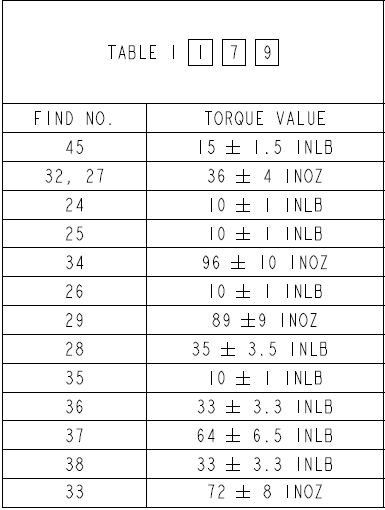## Torque Tolerance

In general, what tolerance range is commonly applied to fastener torque values on drawings?

Expand Hint
Torque (also called moment or couple) is the rotational equivalent of a linear force. It is the force that tends to produce torsion around an axis.
$$\tau = r \times F$$$where $$F$$ is the force, $$\tau$$ is the torque, and $$r$$ is the distance between where the force is applied and where the torque is measured. Hint 2 An example of a fastener torque table from an engineering drawing:Torque (also called moment or couple) is the rotational equivalent of a linear force. It is the force that tends to produce torsion around an axis. $$\tau = r \times F$$$
where $$F$$ is the force, $$\tau$$ is the torque, and $$r$$ is the distance between where the force is applied and where the torque is measured.

Due to calibration range standards, torque wrench tooling imperfections, and inaccuracies with human operation, it is common practice to apply a ±10% tolerance to fastener torque requirement. An example of a fastener torque table from an engineering drawing:±10%